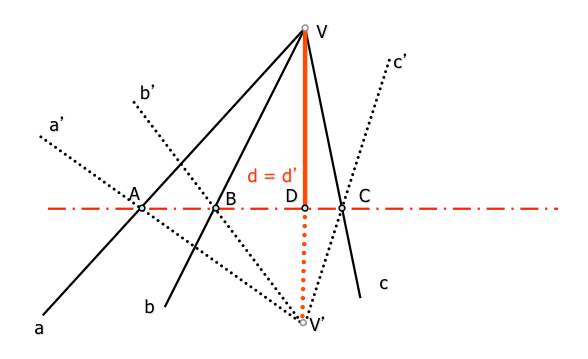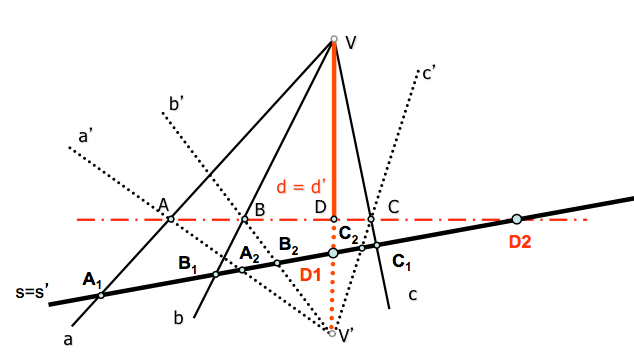# Geometría proyectiva: Overlapping shapes first orderProjective overlapping shapes are a special case of projective shapes, you relate elements of the same type that share a common base.

For example, two overlapping series will have the same line as the basis of geometric shapes, two beams of the same vertex straight (concentric bundles) and two beams overlapping planes around the same axis (coaxiales).

Work mainly with series of dots and straight beams but, everything is developed for these forms, We can generalize flat beams.

On each of the elements of the base elements belonging to find different two ways:

• In an overlapping series have points of the two sets, s1 y s2.
• In a concentric ray beam ….

These elements have their respective counterparts where appropriate, not coincide in general position, except involutions, as seen in Figure.

From an operational point of view, homologous elements for, we can separate the forms or, by projective operations, relate with other second-order; in particular use the circles as projective tools to operate and do overlapping series.

### Overlapping series

We have seen the basic relationships between do pespectivos. These forms share a line called “perspectival axis” beams.If we cut a straight both beams to obtain two overlapping series are projective to each other to be perspectival sections of two beams.The figure shows that this series may have a double colon:

• D1 : Intersection of the base ray with double d = d’ beams perspectival
• D2: Intersection of the base with the perspective axis

### Overlapping beams

We have seen the basic relationships between 'd pespectivas. These forms share a point called “center perspective” series.If we project from a series both obtain two overlapping beams (concentric) which are projective to each other to be screening of two perspectives series.The figure shows that these beams can have two double rays:

• d1 : projection from the base of the double point D = D’ perspectives series
• d2: projection from the base of the perspective center